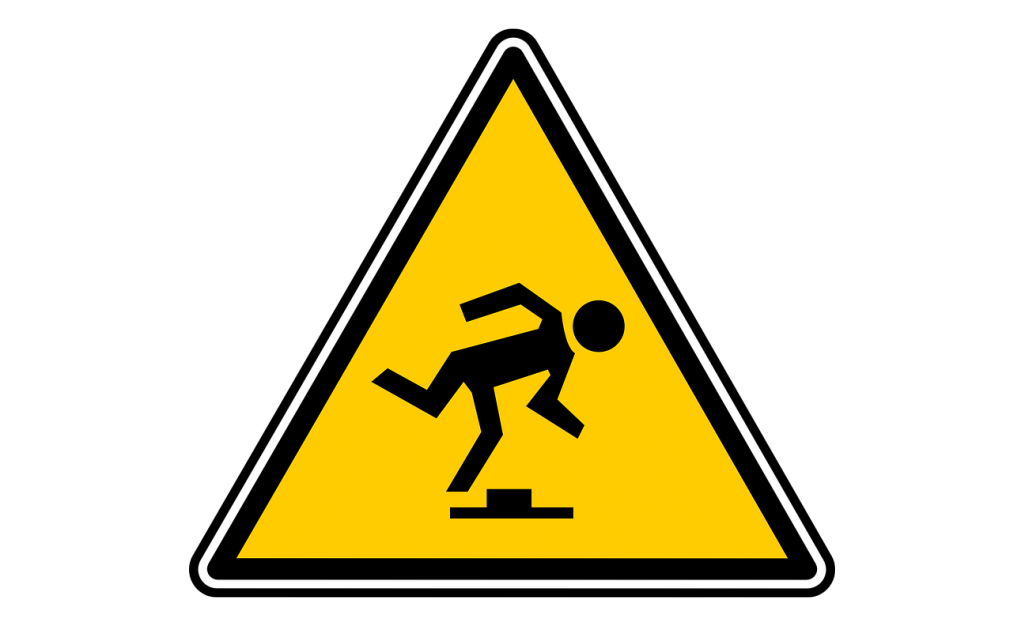# Common Controls

The following controls appear frequently amongst many units.

## V/oct

This parameter is used to transpose the base frequency up or down via a pitch CV (i.e. a signal that is calibrated to 1V/oct, typically one of the inputs from the ABCD matrix). In other words,

$\text{f} = \text{f0} * 2 ^{\frac{x}{1200}}$

where

• $$x$$ is the value of this control
• $$f0$$ is the base frequency (set via the f0 control)
• $$f$$ is the final output frequency of the oscillator

For example, if the base frequency (f0) is set to 55Hz and this V/oct parameter is set to 2400¢, then the resulting frequency will be 220Hz or 2 octaves above 55Hz:

$220\text{Hz} = 55\text{Hz} * 2 ^{\frac{2400}{1200}}$

Modulating this parameter corresponds to exponential FM.

## f0

This parameter sets the fundamental frequency that is subsequently transposed by the V/oct parameter. Modulating this parameter corresponds to (thru-zero) linear FM.

## phase

Sets the phase offset of the oscillator. Modulating this parameter is equivalent to PM (phase modulation). You can even set the f0 parameter to zero (i.e. stop oscillations) and put the oscillator completely under manual control via this phase parameter. This would corresponds to a kind of waveshaping of the phase modulation signal.

## sync

A trigger into this parameter will cause the oscillator to reset its phase accumulator to the value of the phase parameter. This is equivalent to the hard-sync on many oscillators.This sync operation is not anti-aliased. So audio-rate triggering of this parameter will exhibit strong anti-aliasing artifacts which depending on the desired result could be a good thing or a bad thing.

## level

This parameter sets the output gain of the unit (i.e. amplitude modulation).